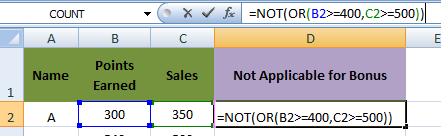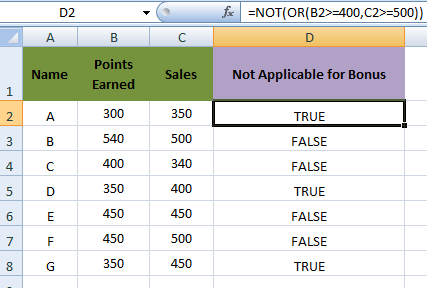### Logical Functions in MS Excel

Logical Functions allows to introduce decision making while executing the functions. Functions are used to check if a condition is true or false.

• AND
• OR
• NOT

### Let's Learn , How to use Logical Functions in MS Excel

How to use IF function
MS Excel IF function returns one value if the condition is TRUE, or another value if the condition is FALSE.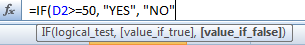Let's learn with Example
The IF function checks whether a condition is met, and returns one value if the condition is TRUE, or another value if the condition is FALSE.
For example, if D2 is less than or equal to 40 then admissions are open , otherwise Admissions are not openso E2 equals =IF(D2<=40, "YES", "NO")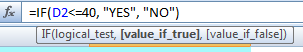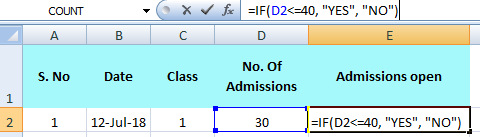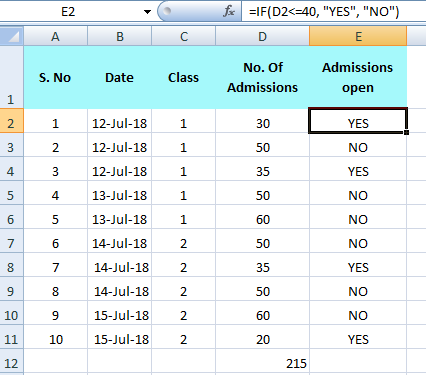How to use AND function
When using the AND function, all conditions within the AND function must be TRUE for the condition to be met. The AND function can be combined with IF function to allow testing for multiple conditions.
Let's learn with Example
The AND Function returns TRUE if all conditions are true and returns FALSE if any of the conditions are false.
For example, if B2 (points earned) are greater than or equal to 400, and C2 (Sales) are greater than or equal to 500, then only person is applicable for bonus, otherwise bonus is not applicable.
So here D2 equals =AND(B2>=400,C2>=500)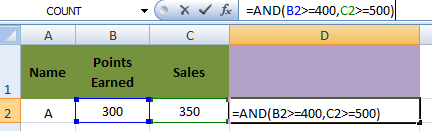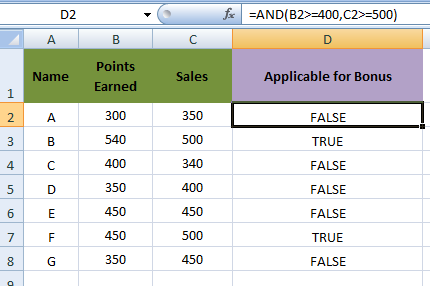How to use OR function
Let's learn with Example
The OR function returns TRUE if any of the conditions are TRUE and returns FALSE if all conditions are false.
For example, in cell D2 Bonus is applicable if, B2 (points earned) are greater than or equal to 400, or C2 (Sales) are greater than or equal to 500.
So here , D2 equals =AND(B2>=400,C2>=500)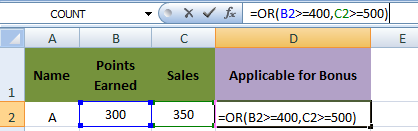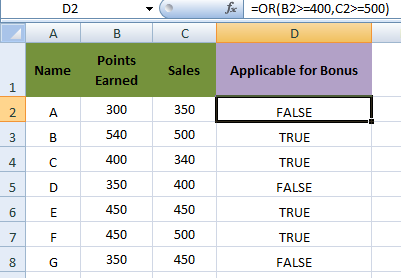How to use NOT function
Let's learn with Example
The NOT function changes TRUE to FALSE, and FALSE to TRUE.
For example, in cell D2 Bonus is Not applicable if, B2 (points earned) are greater than or equal to 400, or C2 (Sales) are greater than or equal to 500.
So here , D2 equals =NOT(OR(B2>=400,C2>=500))